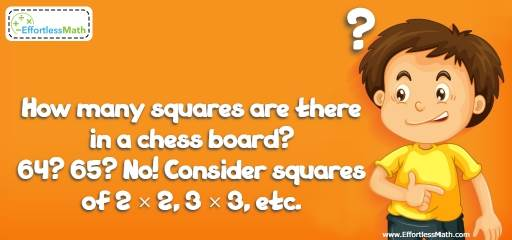# Number Properties Puzzle -Critical Thinking 1

Love to solve complicated math and critical thinking challenges? If so, you are in the right place! It takes a genius to solve this tricky critical-thinking puzzle!## Challenge:

How many squares are there in a chess board?
64? 65? No! Consider squares of 2 × 2, 3 × 3, etc.

### The Absolute Best Book to challenge your Smart Student!

This question implies “squares of any size”. You can find squares of 1 by 1, 2 by 2, 3 by 3 and so on. A chessboard is a square of 8 by 8 and hence there are 64 (8 x 8 = 64) 1 by 1 square on a chessboard.
The next size square is a 2 by 2 unit square. First, find all 2 by 2 squares in the first two rows. You can find seven of them. Then, consider rows 2 and 3. Again, you can find seven. Consider rows 3-4, 4-5, 5-6, 6-7, and 7-8. You’ll find seven 2 by 2 squares for each pair. Therefore, there are 7 × 7 = 49 squares of 2 by 2.
We can use the same pattern for the squares of 3×3. You can find six squares of 3×3 in the first 3 rows. Then, consider rows of 2-3-4, 3-4-5, and so on. You’ll find 6 × 6 = 36 squares of 3 by 3.
Continue the pattern for other size of square.
64 squares of 1 × 1
49 squares of 2 × 236 squares of 3 × 3
25 squares of 4 × 4
16 squares of 5 × 5
9 squares of 6 × 6
4 squares of 7 × 7
1 square of 8 × 8
Total number of squares:
64 + 49 + 36 + 25 + 16 + 9 + 4 + 1 = 204

The Absolute Best Books to Ace Algebra

### What people say about "Number Properties Puzzle -Critical Thinking 1 - Effortless Math: We Help Students Learn to LOVE Mathematics"?

No one replied yet.

X
45% OFF

Limited time only!

Save Over 45%

SAVE $40 It was$89.99 now it is \$49.99